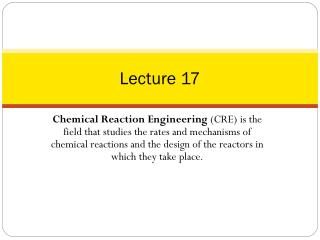# Lecture 17 - PowerPoint PPT PresentationDownload PresentationLecture 17

Lecture 17Download Presentation## Lecture 17

- - - - - - - - - - - - - - - - - - - - - - - - - - - E N D - - - - - - - - - - - - - - - - - - - - - - - - - - -
##### Presentation Transcript

1. Lecture 17 Chemical Reaction Engineering (CRE) is the field that studies the rates and mechanisms of chemical reactions and the design of the reactors in which they take place.

2. Today’slecture • Energy Balance Fundamentals • Adibaticreactors

3. Energy Balances, Rationale and Overview TodaysLecture Let’s calculate the volume necessary to achieve a conversion, X, in a PFR for a first-order, exothermic and adiabatic reaction. The temperature profile might look something like this: T k X V V V

4. Energy Balances, Rationale and Overview The combinedmolebalance, rate law and stoichiometryyield:

5. Energy Balances, Rationale and Overview Wecannotsolve this Equationbecausewedon’thave X either as a function of V or T. WeneedanotherEquation. That Equation is: The Energy Balance

6. User Friendly Equations Relate T and X or Fi 1. Adiabatic CSTR, PFR, Batch or PBR

7. Adiabatic T T Exothermic Endothermic XEB XEB T0 T0 0 0

8. User Friendly Equations Relate T and X or Fi 2. CSTR with heat exchanger, UA(Ta-T) and a large coolant flow rate T Ta

9. User Friendly Equations Relate T and X or Fi 3. PFR/PBR with heat exchange Coolant Ta FA0 T0 3A. PFR in terms of conversion

10. User Friendly Equations Relate T and X or Fi 3B. PBR in terms of conversion 3C. PBR in terms of molar flow rates

11. User Friendly Equations Relate T and X or Fi 3D. PFR in terms of molar flow rates 4. Batch

12. User Friendly Equations Relate T and X or Fi 5. For Semibatch or unsteady CSTR 6. For multiple reactions in a PFR (q reactions and m species) Let’s look wheretheseUserFriendlyEquationscame from.

13. Energy Balance Rate of energy in by flow Rate of energy out by flow Heat added to the system Work done by the system Rate of energy accumulation - + - =

14. Energy Balance Energy Balance on an open system: schematic.

15. OK folks, here is whatwe are going to do to put the aboveequationinto a usable form. 1. ReplaceUi by Ui=Hi-PVi 2. Express Hi in terms of heat capacities 3. Express Fi in terms of eitherconversion or rates of reaction 4. DefineΔHRx 5. Define ΔCP 6. Manipulate so that the overall energybalance is either in terms of the UserFriendlyEquations.

16. Intro to Heat Effects Assumptions: =0 =0 Other energies small compared to internal Recall:

17. Intro to Heat Effects Substituting for

18. Intro to Heat Effects General Energy Balance: For Steady State Operation:

19. Intro to Heat Effects For the generalizedreaction: Flow Rates, Fi In general,

20. Intro to Heat Effects

21. Intro to Heat Effects For No Phase Changes Constant Heat Capacities Enthalpy of formation at temperature TR Heat of reaction at temperature T

22. Intro to Heat Effects

23. Intro to Heat Effects

24. Intro to Heat Effects Substituting back into the Energy Balance

25. T Adiabatic Energy Balance: T0 X

26. ExampleAdiabatic PFR A ↔ B 1) Mole balance: 2) Rate Laws:

27. ExampleAdiabatic PFR A ↔ B 3) Stoichiometry: 4) Energy Balance: First need to calculate the maximum conversionwhich is at the AdiabaticEquilibrium.

28. ExampleAdiabatic PFR A ↔ B XC Adiabatic equilibrium conversion T

29. Wecannow form a table. Set X, thencalculate T, -VA, and FA0/-rA, increment X, thenplot FA0/-rA vs. X: FA0/-rA X

30. End of Lecture 17